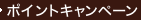•### Numbers and Functions : Steps into Analysis

• ただいまウェブストアではご注文を受け付けておりません。 ⇒古書を探す
• 製本 Hardcover:ハードカバー版
• 言語 ENG,ENG
• 商品コード 9780521410861
• DDC分類 515.7

### Table of Contents

`IntroductionPart I. Numbers: 1. Counting numbers andmathematical induction2. Order, arithmetic with inequalities3. Sequences, a first bite at infinity4. Completeness, what the rational numbers lack5. Series, infinite sumsPart II. Functions: 6. Functions andcontinuity: neighbourhoods, limits of functions7. Continuity and completeness, functions onintervals8. Derivatives, tangents9. Differentiation and completeness: mean valuetheorems10. Integration: the fundamental theorems,Taylor's theorem11. Indices and circle functions12. Sequences of functions.`